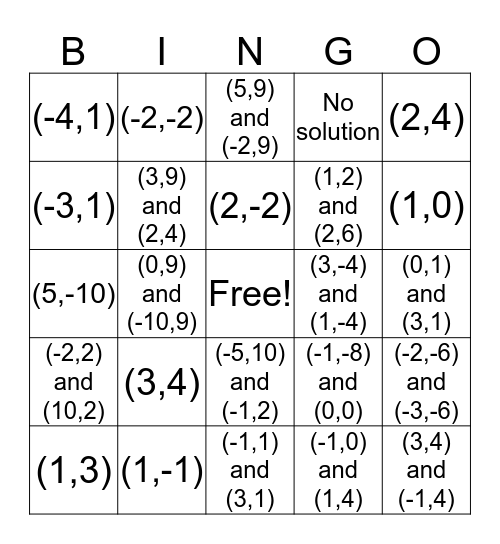Solving Systems by GraphingThis bingo card has a free space and 24 words: (3,4), (1,-1), (2,-2), (1,3), (2,4), (-4,1), (-3,1), (-2,-2), (3,-4) and (1,-4), (-5,10) and (-1,2), (1,2) and (2,6), (5,-10), (-1,-8) and (0,0), (-2,-6) and (-3,-6), (3,9) and (2,4), (-1,0) and (1,4), (0,1) and (3,1), (5,9) and (-2,9), (1,0), (0,9) and (-10,9), (3,4) and (-1,4), (-2,2) and (10,2), No solution and (-1,1) and (3,1).

Play Online